﻿ 电容电导传感器油水两相流含率测量
 中国科学院大学学报2017, Vol. 34Issue (2): 237-243PDF

Capacitance-conductance sensor for water holdup measurement in oil-water two-phase flow
WU Hao, TAN Chao, DONG Feng
Tianjin Key Laboratory of Process Measurement and Control, School of Electrical Engineering and Automation, Tianjin University, Tianjin 300072, China
Abstract: In oil-water two-phase flow measurement, a capacitance-conductance sensor has been designed based on the fact that the capacitance sensor and conductance sensor realize the water holdup measurement in different situations with different sensitive ranges. Electrical switches are used to switch the two measurement methods at high speed in order to make them have the same sensitive fields. The disigned sensor realizes the whole fraction measurement in oil-water two-phase flow with low conductivity water (tap water) and the water holdup measurement error is less than 5[WTB4]%[WTBZ].
Key words: oil-water two-phase flow     capacitance-conductance sensor     water holdup measurement     sensor optimization

1 电容电导传感器结构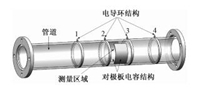Download: JPG larger image 图 1 电容电导传感器结构 Fig. 1 Structure of the capacitance-conductance sensor

2 理论模型

2.1 电导测量模型

 $V = \frac{{{V_{\text{w}}}}}{{{V_{\text{m}}}}},$ (1)

 ${\sigma _{\text{m}}} = \frac{{2{H_t}}}{{3 - {H_t}}}{\sigma _{\text{w}}},$ (2)

 ${H_t} = \frac{{3V}}{{2 + V}}.$ (3)

2.2 电容测量模型

 ${\text{RCD}} = \frac{{{C_{\text{m}}} - {C_{\text{o}}}}}{{{C_{\text{w}}} - {C_{\text{o}}}}} = \frac{{{V_{{\text{cm}}}} - {C_{{\text{co}}}}}}{{{V_{{\text{cw}}}} - {V_{{\text{co}}}}}},$ (4)

(a) 当管道内充满导电水时，可以认为是容性负载和阻性负载并联，设RwCw1分别是导电水的电阻值和电容值，其阻抗可表示为

 ${Z_{{\text{w1}}}} = \frac{2}{{{\text{j}}\omega {C_{\text{p}}}}} + \frac{{{R_{\text{w}}}}}{{1 + j\omega {C_{{\text{w1}}}}{R_{\text{w}}}}}.$ (5)

(b) 当管道内充满非导电水时，可以认为只有容性负载，设Cw2为非导电水的电容值，其阻抗可以表示为

 ${Z_{{\text{w2}}}} = \frac{2}{{{\text{j}}\omega {C_{\text{p}}}}} + \frac{1}{{1 + j\omega {C_{{\text{w2}}}}}}.$ (6)

Vcm与极板之间的电容值大小成正比，定义一个参数γ=|1/Zw1|/|1/Zw2|，代入方程 (4)，得到修正的RCD值

 ${\text{RC}}{{\text{D}}^ * } = \frac{{{V_{{\text{cm}}}} - {C_{{\text{co}}}}}}{{{V_{{\text{cw}}}}/\gamma - {V_{{\text{co}}}}}}.$ (7)

3 电容电导传感器测量系统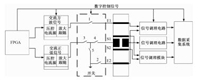Download: JPG larger image 图 2 电容电导传感器测量系统结构框图 Fig. 2 Structure diagram of the capacitance-conductance sensor measurment system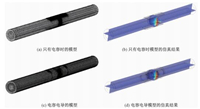Download: JPG larger image 图 3 电容传感器模型与电容电导传感器模型仿真结果 Fig. 3 Simulation results of the capacitance sensor model and the conductance-capacitance sensor model

4 电容电导传感器油水两相流实验 4.1 电容电导传感器层流响应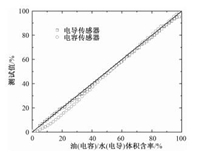Download: JPG larger image 图 4 电容电导传感器层流响应 Fig. 4 Stratified flow response to the capacitance-conductance sensor
4.2 实验装置介绍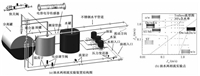Download: JPG larger image 图 5 油水两相流实验装置结构图及实验点设计 Fig. 5 Structure diagram of the oil-water two-phase flow experimental device and the experimental condition
4.3 电容电导传感器动态标定实验

 ${E_r} = \frac{{{\text{Holdu}}{{\text{p}}_{{\text{meas}}}} - {\text{Holdu}}{{\text{p}}_{{\text{QCV}}}}}}{{{\text{Holdup}}\;{\text{range}}}} \times 100\% ,$ (8)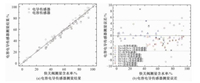Download: JPG larger image 图 6 电容电导传感器测量结果与快关阀结果对比 Fig. 6 Measurement result comparison between the capacitance-conductance sensor and QCV

5 结论

  Xie C G, Reinecke N, Beck M S, et al. Electrical tomography techniques for process engineering applications[J]. The Chemical Engineering Journal and the Biochemical Engineering Journal, 1995, 56(3):127–133. DOI:10.1016/0923-0467(94)02907-5  Zhao X, Lucas G P. Use of a novel dual-sensor probe array and electrical resistance tomography for characterization of the mean and time-dependent properties of inclined, bubbly oil-in-water pipe flows[J]. Measurement Science and Technology, 2011, 22(10):104012. DOI:10.1088/0957-0233/22/10/104012  Ismail I, Gamio J C, Bukhari S F, et al. Tomography for multi-phase flow measurement in the oil industry[J]. Flow Measurement and Instrumentation, 2005, 16(2):145–155.  Dong F, Liu X P, Deng X, et al. Identification of two-phase flow regimes in horizontal, inclined and vertical pipes[J]. Measurement Science and Technology, 2001, 12(8):1069–1075. DOI:10.1088/0957-0233/12/8/312  Fossa M. Design and performance of a conductance probe for measuring the liquid fraction in two-phase gas-liquid flows[J]. Flow Measurement and Instrumentation, 1998, 9(2):103–109. DOI:10.1016/S0955-5986(98)00011-9  Devia F, Fossa M. Design and optimisation of impedance probes for void fraction measurements[J]. Flow Measurement and Instrumentation, 2003, 14(4):139–149.  Dang R R, Zhao D S, Li L P, et al. The flow measurement of multiphase flow based on the conductance sensor[J]. Modern Instrumentation, 2012, 1(4):35–40. DOI:10.4236/mi.2012.14005  Abouelwafa M S A, Kendall E J M. The use of capacitance sensors for phase percentage determination in multiphase pipelines[J]. IEEE Transactions on Instrumentation and Measurement, 1980, 29(1):24–27. DOI:10.1109/TIM.1980.4314856  Jaworek A, Krupa A. Gas/liquid ratio measurements by RF resonance capacitance sensor[J]. Sensors and Actuators A:Physical, 2004, 113(2):133–139. DOI:10.1016/j.sna.2004.02.006  Reis E D, Cunha D S. Experimental study on different configurations of capacitive sensors for measuring the volumetric concentration in two-phase flows[J]. Flow Measurement and Instrumentation, 2014, 37:127–134. DOI:10.1016/j.flowmeasinst.2014.04.001  Wu H, Tan C, Dong X, et al. Design of a conductance and capacitance combination sensor for water holdup measurement in oil-water two-phase flow[J]. Flow Measurement and Instrumentation, 2015, 46(B):218–229.  施艳艳, 董峰, 谭超. 两相流测量中环形电导传感器特性研究[J]. 中国电机工程学报, 2010, 30(17):62–66.  董虓霄, 谭超, 董峰. 油水两相流含水率测量组合传感器[J]. 工程热物理学报, 2015, 36(7):1487–1491.  Yang W Q. Hardware design of electrical capacitance tomography systems[J]. Measurement Science and Technology, 1996, 7(3):225–232. DOI:10.1088/0957-0233/7/3/003  Trallero J L, Sarica C, Brill J P. A study of oil/water flow patterns in horizontal pipes[J]. SPE Production & Facilities, 1997, 12(3):165–172.